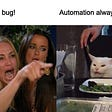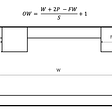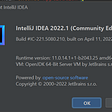# Extending the range of integers with no-exponent posits

Posits were proposed as a replacement for standard floating-point numbers. Can they replace standard integers too?

In this piece I will discuss about when this might make sense.

# Anatomy of a no-exponent posit

A posit number is mainly composed of three parts: the regime, the exponent and the significand.

The regime is a run of identical-value bits followed by an end bit. The exponent is a fixed-length value placed after the regime. The rest of the bits make up the significand.

Example: 11001101111

regime: 11(0)
exponent: 01
significand: 101111

Because computers work with fixed bit-length values, posit numbers are truncated to a particular bit length. Any truncated bit is assumed to be zero.

Posits are usually signed. They use the two’s-complement scheme for negative numbers and the negative number without an opposite (INT_MIN) is used to represent infinity or an invalid value.

The length of the exponent is decided in advance; the latest posit standard settles on a 2-bit exponent.

For integers we will want a zero-bit exponent. This means that the significand follows immediately the regime bits, with no middle bits reserved for the exponent. In that case the value of the regime is where r=n-1 for a run of n ones and r=-n for a run of n zeros.

The significand works in the same way as standard floating-point numbers: it represents the fractional part of a binary real number which integer part is implicitely 1. So the first bit of the significand represents 0 or 1/2, the second bit represents 0 or 1/4, the third bit 0 or 1/8 etc. For example if the significand is 101, that corresponds to the number 1.101 in binary which is 1+1/2+0+1/8 = 1.625 in decimal.

Since we have no exponent part, that is just multiplied by the value of the regime to give a number. But why am I talking at length about floating-point numbers when the title of the article is all about integers?

# Posits to integers

It turns out that no-exponent posits are equivalent to successive integers divided by a fixed number for half of their possible values. I will demonstrate this with an example. I am taking 5-bit posits (ignoring the sign); in that case the number to divide by is 16. The last column in the table below shows which integer is divided by 16 to give a posit value:

What do you see? Usually five bits are able to represent integers from 0 to 31; here we go up to 256, which usually requires about eight bits! But after the midpoint (16) there are gaps between successive values which widen progressively.

That is good and that is bad. It is good that the range of integers that can be represented in the same number of bits increases; it is bad that there are discontinuities in the range.

Some numbers that can be represented with standard integers are missing (odd values greater than 16). Also some calculations may no longer work — for example, let us consider the following pseudo-code:

For n = 0; n < 100; n ← n+1:
Printline(n)
End

This will never stop printing “16” when using the integers we defined above: the operation n+1 cannot give 17 since that integer is missing from the table. The value is rounded to 16 and n ← n+1 has no effect.

The way to work around this would be to use an integer range as source of the loop variable instead of advancing it manually by one for each iteration. More generally, the programmer needs to be aware that not all values in a range can be represented.

# Comparison with standard integers

Since the first half of standard integers relate directly to their posit-powered counterparts, the cost of extending the range of integers is one bit. For example standard signed 32-bit integers can represent all numbers between -2³¹ and 2³¹-1 (2,147,483,647). The posit-based ones will represent all numbers between -2³⁰ and 2³⁰ (1,073,741,824).

On the other hand the maximum value will be 2⁶¹, which is close in range to standard 64-bit integers!

Similarly, posit-based 64 bit integers can represent all numbers up to 2⁶² (4,611,686,018,427,387,904) and the range extends to 2¹²⁵, which is close to standard 128-bit integers.

Hardware performance of standard integer operations is usually better; actually modern processors can perform floating point operations very fast too. Unfortunately there is little hardware support for posit numbers today. This could change in the future as posit adoption increases.

Hardware support for posit-based integers should require little deviation from normal posit support. It is possible some manufacturers could decide to include both which would help performance tremendously.

Posits are designed as replacement for standard floating point numbers. In some cases, they can replace standard integer numbers too:

• The numbers are rarely close to the limit of the standard integer range
• When they come close to that limit, they do not require full precision
• It would be helpful to extend that range further at the cost of some precision
• Arithmetic performance does not matter much or the hardware supports posits

If you liked that story or want to discuss it, please comment below!

--

--

--

## More from Denis Bredelet

Love podcasts or audiobooks? Learn on the go with our new app.

## Recommended from Medium## Kunth-Morris-Pratt(KMP) Algorithm For Pattern Searching## How To Deploy Java APIs on GCP GKE using HELM## Why I Use DMXzone as a Framework (And You Should, Too!)## Web3Games Community AMA-中文## TestNG. Life without XML: handling flaky tests.## New Neovim Completion Plugins You Should Try## Transistors and the Legend of Zelda## Convolution Layer with Numpy## [Solved] Error: JavaFX runtime components are missing, and are required to run this application## Connor C